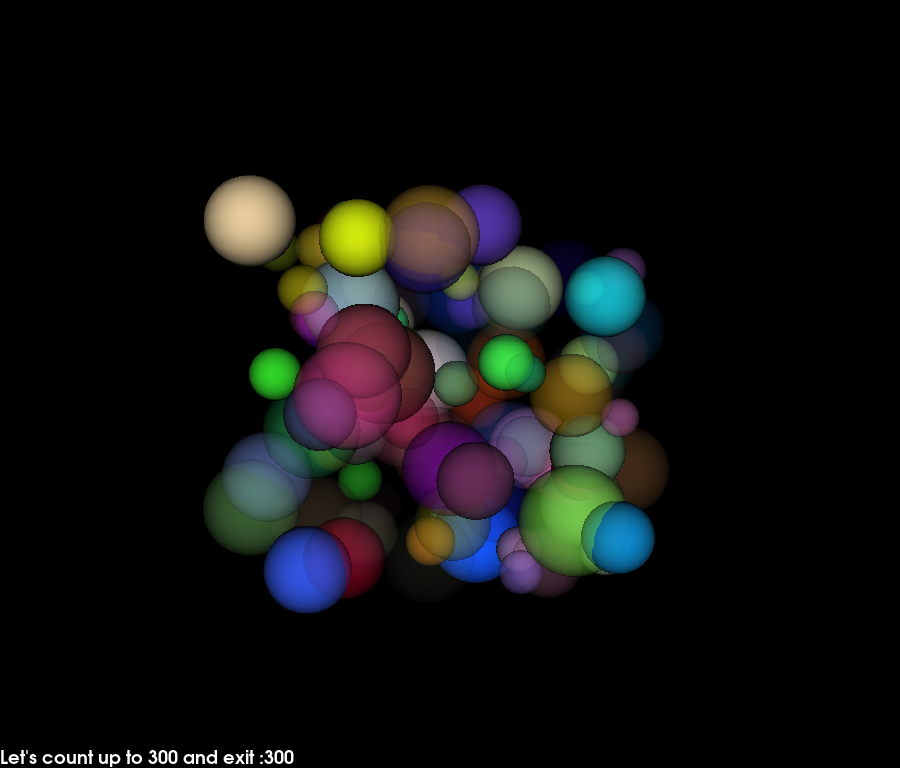# Using a timer#

This example shows how to create a simple animation using a timer callback.

We will use a sphere actor that generates many spheres of different colors, radii and opacity. Then we will animate this actor by rotating and changing global opacity levels from inside a user defined callback.

The timer will call this user defined callback every 200 milliseconds. The application will exit after the callback has been called 100 times.```import itertools

import numpy as np

from fury import actor, ui, window

xyz = 10 * np.random.rand(100, 3)
colors = np.random.rand(100, 4)

scene = window.Scene()

showm = window.ShowManager(
scene, size=(900, 768), reset_camera=False, order_transparent=True
)

tb = ui.TextBlock2D(bold=True)

# use itertools to avoid global variables
counter = itertools.count()

def timer_callback(_obj, _event):
global timer_id
cnt = next(counter)
tb.message = "Let's count up to 300 and exit :" + str(cnt)
showm.scene.azimuth(0.05 * cnt)
sphere_actor.GetProperty().SetOpacity(cnt / 100.0)
showm.render()

if cnt == 10:
# destroy the first timer and replace it with another faster timer
showm.destroy_timer(timer_id)

if cnt == 300:
# destroy the second timer and exit
showm.destroy_timer(timer_id)
showm.exit()